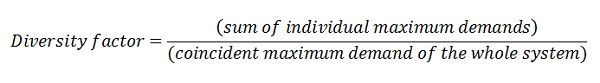# Diversity Factor

Definition: Diversity factor is defined as the ratio of the sum of the maximum demands of the various part of a system to the coincident maximum demand of the whole system. The maximum demands of the individual consumers of a group do not occur simultaneously. Thus, there is a diversity in the occurrence of the load. Due to this diverse nature of the load, full load power supply to all the consumers at the same time is not required.Mathematically, diversity factor is expressed  as:

FD – diversity factor
Di – maximum demand of the load I, irrespective of the time of occurrence.
Dg = D(1+2+3…….n) – maximum coincident demands of a group of n load.

Diversity factors can be defined for loads, substations, feeders, and generating stations. Usually, the maximum demands of the consumers do not occur at the same time. The diversity factor can be equal or greater than 1.

If the value of the diversity factor is greater than 1, then it is a good diversity factor, and 1.0 represents a poor diversity factor. A high diversity factor has the effect of reducing the maximum demand. It is obtained by using electrical energy at night load or light load periods.

### 7 thoughts on “Diversity Factor”

1. Please provide an example calculation.
If
Maximum Demand of Load 1 = 10KVA,
Maximum Demand of Load 2 = 20KVA,
Maximum Demand of Load 3 = 30KVA
and
Maximum Demand of the Total System = 50KVA
then
Diversity Factor = (10+20+30) : 50 = 60:50 = 1.2:1